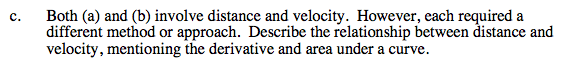### Home > CALC > Chapter 4 > Lesson 4.2.2 > Problem4-58

4-58.
1.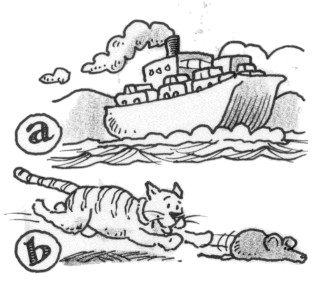Compare how distance and velocity are related with these two scenarios: Homework Help ✎

1. A ferry crosses the bay so that its distance (in miles) from the dock at time t is d(t) = 1 −cos t. Find the velocity, d ′(t), at times t = 1, π, and 5 hours. Explain what concepts of calculus you applied in order to solve this problem.

2. When a cat chases a mouse, the cat's velocity, measured in feet per second, is v(t) = 3t. Sketch a graph and find the distance the cat ran in the first 5 seconds. Explain what concepts of calculus you applied in order to solve this problem.

3. Both (a) and (b) involve distance and velocity. However, each required a different method or approach. Describe the relationship between distance and velocity, mentioning the derivative and area under a curve.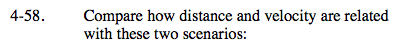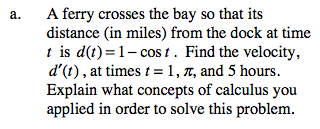Explain WHY d '(t) = v(t). Describe what velocity looks like on a distance graph.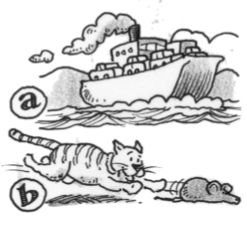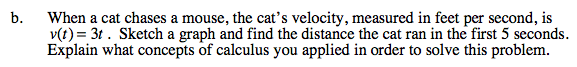Describe what distance (or displacement) looks like on a velocity graph.